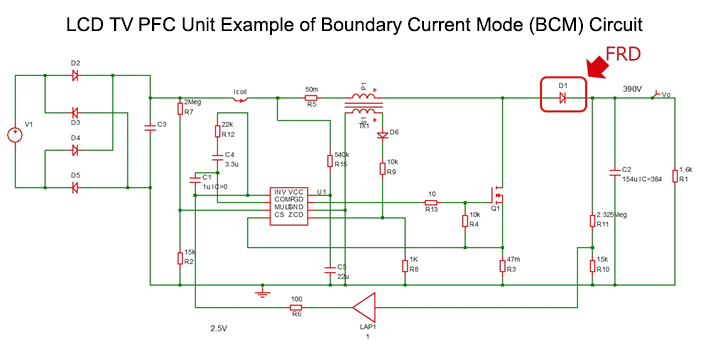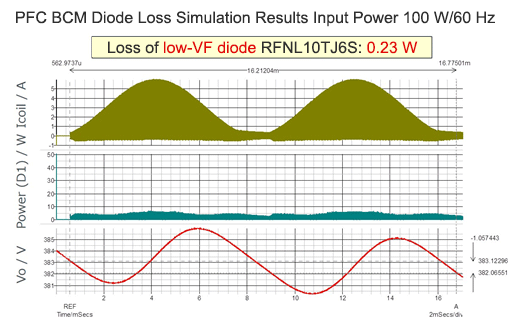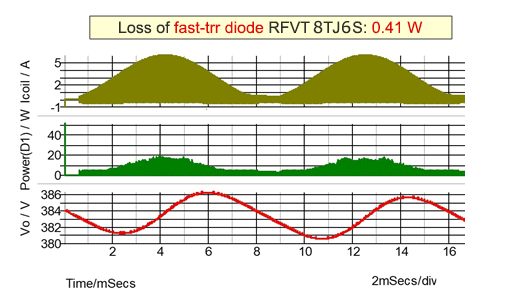Technical Information Site of Power Supply Design

2018.08.23 Si Power Device

# Boundary Current Mode PFC：Examples of Efficiency Improvement Using Diodes

Application Examples that Exploit Feature

In actual application circuits, diodes and transistors must be used selectively according to differences in their characteristics and performance. The main objective of selective use in power applications is to improve efficiency. In this article, we present an example of efficiency improvement in boundary current mode PFC (power factor correction) through differences in diode characteristics. Regarding PFC, see the explanation in the previous article.

Boundary Current Mode PFC: Example of LCD TV Circuit

This circuit is the PFC section of an LCD TV, and is an example of a single PFC boundary current mode (BCM) control method. The PFC circuit diode D1 is a fast-recovery diode (hereafter "FRD").Loss simulations were performed for cases in which, a diode with low forward voltage VF, and a diode with a fast reverse recovery time trr were used. The main specifications of the two types of diode used are shown below. Specifications other than VF and trr were essentially the same.

RFNL10TJ6S Low VF RFV8TJ6S Fast trr Unit
VRM 600 600 V
IF 10 8 A
VF 1.25 @IF=8A 3 @IF=8A Vmax
IR 10 10 µA
trr 65 20 ns max

The simulation results for each case were as follows.The waveforms in the center represent the diode loss power. The upper waveforms are the coil currents which are the diode currents IF, and the lower waveforms are the output voltage, or the voltage applied to the diodes. The diode loss power is as shown by the waveforms, and is lower for the low-VF diode RFNL10TJ6S. Average loss values are 0.23 W for the RFNL10TJ6S with low VF and standard trr, and 0.41 W for the RFV08TJ6S with fast trr and standard VF. The difference in VF values is 1.25 V versus 3 V (when IF = 8 A).

These results indicate that in boundary current mode PFC, differences in the diode VF have a major effect on losses, whereas the effect of trr is small. This is because in boundary current mode, currents from zero to peak value flow, and so if the diode VF is high, to this extent conduction losses will be increased.

In PFC under boundary current mode control, by selecting a diode with a low a VF as possible, circuit efficiency can be improved.

In the next article, we will explain the effect of diodes in continuous current mode (CCM).

#### Key Points:

・In boundary current mode PFC, the diode VF has a major effect on losses, whereas the effect of the diode trr is small.

・In PFC under boundary current mode control, by selecting a diode with a low VF, circuit efficiency can be improved.